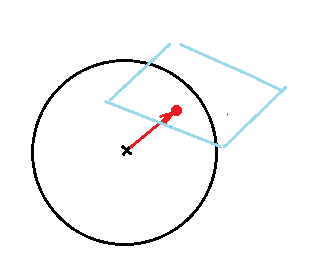# Find intersections between sphere and parallel tangent planes

Mod note: Moved from a technical forum section, so missing the homework template.
@fab13 -- please post homework problems in the appropriate section under Homework & Coursework.

I have the following exercise to solve : I have to find all the points on the surface ##x^2+y^2+z^2=36## (so a sphere of radius = 6) where tangent planes are parallel to plane ##3x+4y+5z=0##

For the moment, I can get this reasoning :

if ##M_{0}=(x_0,y_0,z_0)## is a point that staisfies the wanted property above and ##(x,y,z)## a point of its tangent plane, I have (with ##C## the center of the sphere) :

$$\vec{CM_{0}}\cdot \vec{MM_{0}}=0$$

So with the sphere of equation : ##(x-a)^2+(y-b)^2+(z-c)^2=R^2##, I can deduce :

##(x_0-a)(x-x_0)+(y_0-b)(y-y_0)+(z_0-c)(z-z_0)=0## and especially :

##(x_0-a)(x-a)+(y_0-b)(y-b)+(z_0-c)(z-c)-R^2=0##

In my case, ##R^2=36## and ##a=b=c=0##, so I have :

##x_0 x +y_0 y +z z_0 -36=0##

From this point, how can I deduce ##(x_0,y_0,z_0)## ( I recall, the tangent plane at this point has to be parallel to plane ##3x+4y+5z=0##) ?

Last edited by a moderator:

ehild
Homework Helper
Mod note: Moved from a technical forum section, so missing the homework template.
@fab13 -- please post homework problems in the appropriate section under Homework & Coursework.

I have the following exercise to solve : I have to find all the points on the surface ##x^2+y^2+z^2=36## (so a sphere of radius = 6) where tangent planes are parallel to plane ##3x+4y+5z=0##

For the moment, I can get this reasoning :

if ##M_{0}=(x_0,y_0,z_0)## is a point that staisfies the wanted property above and ##(x,y,z)## a point of its tangent plane, I have (with ##C## the center of the sphere) :

$$\vec{CM_{0}}\cdot \vec{MM_{0}}=0$$

So with the sphere of equation : ##(x-a)^2+(y-b)^2+(z-c)^2=R^2##, I can deduce :

##(x_0-a)(x-x_0)+(y_0-b)(y-y_0)+(z_0-c)(z-z_0)=0## and especially :

##(x_0-a)(x-a)+(y_0-b)(y-b)+(z_0-c)(z-c)-R^2=0##

In my case, ##R^2=36## and ##a=b=c=0##, so I have :

##x_0 x +y_0 y +z z_0 -36=0##

From this point, how can I deduce ##(x_0,y_0,z_0)## ( I recall, the tangent plane at this point has to be parallel to plane ##3x+4y+5z=0##) ?

You know that the radius of the sphere drawn to the tangent point is normal to the tangent plane.
What is the normal of the tangent plane?#### Attachments

Last edited:
the vector ##\vec{V}=(3,4,5)## is normal to plane ##3x+4y+5z=0##

So, by a proportional rule, I mean, for example ##x_0## :

## \sqrt{50} \rightarrow 3##
##6 \rightarrow ???##

can I take ##x_0=18/\sqrt{50}=9\sqrt{2}/5##, ##y_0=24/\sqrt{50}=12\sqrt{2}/5##, ##z_0=3\sqrt{2}## ??

ehild
Homework Helper
the vector ##\vec{V}=(3,4,5)## is normal to plane ##3x+4y+5z=0##

So, by a proportional rule, I mean, for example ##x_0## :

## \sqrt{50} \rightarrow 3##
##6 \rightarrow ???##

can I take ##x_0=18/\sqrt{50}=9\sqrt{2}/5##, ##y_0=24/\sqrt{50}=12\sqrt{2}/5##, ##z_0=3\sqrt{2}## ??
Yes. Good work.

Ray Vickson
Homework Helper
Dearly Missed
the vector ##\vec{V}=(3,4,5)## is normal to plane ##3x+4y+5z=0##

So, by a proportional rule, I mean, for example ##x_0## :

## \sqrt{50} \rightarrow 3##
##6 \rightarrow ???##

can I take ##x_0=18/\sqrt{50}=9\sqrt{2}/5##, ##y_0=24/\sqrt{50}=12\sqrt{2}/5##, ##z_0=3\sqrt{2}## ??
OK, but don't forget the other point on the sphere where the tangent is also parallel to the given plane.

OK, but don't forget the other point on the sphere where the tangent is also parallel to the given plane.
you mean negative values, i.e the symmetric "opposite" point ?

Ray Vickson